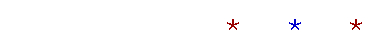Proof of the double-angle and half-angle formulas

Double-angle formulasProof

The double-angle formulas are proved from the sum formulas by putting β =.  We have

 sin 2= sin (+) = sincos+ cossin= 2 sincos. cos 2= cos (+) = coscos− sinsincos 2= cos2− sin2.   .  .  .  .  .  . (1)

This is the first of the three versions of cos 2.  To derive the second version, in line (1) use this Pythagorean identity:

sin2= 1 − cos2.

Line (1) then becomes

 cos 2= cos2− (1 − cos2) = cos2− 1 + cos2. cos 2= 2 cos2− 1.  .  .  .  .  .  .  .  .  .  (2)

To derive the third version, in line (1) use this Pythagorean identity:

cos2= 1 − sin2.

We have

 cos 2= 1 − sin2− sin2;. cos 2= 1 − 2 sin2.  .  .  .  .  .  .  .  .  .  (3)

These are the three forms of cos 2.

Half−angle formulas.  .  .  .  .  .  .  (2').  .  .  .  .  .  .  (3')

Whether we call the variable θ ordoes not matter.  What matters is the form.

Proof

Now,is half of 2.  Therefore, in line (2), we will put 2= θ, so thatbecomes θ2 :
 cos θ = 2 cos2 θ2 − 1.
 On solving this algebraically for cos θ2 , we will have the half-angle

formula for the cosine.

So, on transposing 1 and exchanging sides, we have

 2 cos2 θ2 = 1 + cos θ cos2 θ2 = ½(1 + cos θ) cos θ2 =.

This is the half-angle formula for the cosine.  The sign ± will depend on the quadrant of the half-angle.  Again, whether we call the argument θ ordoes not matter.

Notice that this formula is labeled (2') -- "2-prime"; this is to remind us that we derived it from formula (2).

 The formula for sin θ2 comes from putting 2= θ in line (3).  On

transposing, line (3) becomes

 2 sin2 θ2 = 1 − cos θ, so that sin θ2 =.

This is the half−angle formula for the sine.Trigonometric identities